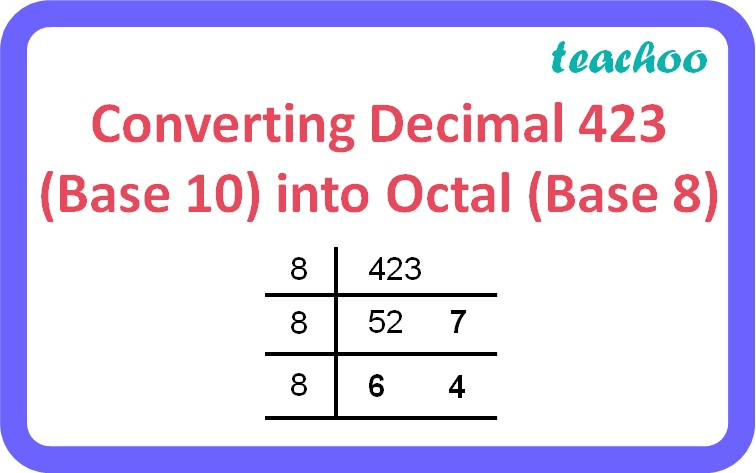3 Mark Questions

Computer Science - Class 11
Chapter 2 Class 11 - Encoding Schemes and Number System

## Convert (423.03125)10 to octal form.

(423.03125) 10 is a decimal number.

423 is the integer part of the number and .03125 is the fractional part of the number.

Step 1: Converting 423 to octal(423) 10 = (647) 8

Step 2: Converting .03125 to octal

 Integer Part Fractional Part 0.03125 x 8 = 0.25 0 ↓ 0.25 0.25 x 8 = 2 2 ↓ 0

(0.03125) 10 = (0.02) 8

Therefore, (423.03125) 10 = (647.02) 8

Learn in your speed, with individual attention - Teachoo Maths 1-on-1 Class

### Transcript

Converting Decimal 423 (Base 10) into Octal (Base 8)